American Journal of Theoretical and Applied Statistics
Volume 6, Issue 5-1, September 2017, Pages: 8-12

A Generalization of Some Lag Synchronization of System with Parabolic Partial Differential Equation

Mahmoud M. El-Borai*, Wagdy G. El-sayed., Aafaf E. Abduelhafid

Department of Mathematics and Computer Science, Faculty of Science, Alexandria University, Alexandria, Egypt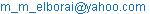(M. M. El-Borai)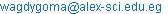. (W. G. El-sayed)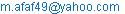(A. E. Abduelhafid)

*Corresponding author

Mahmoud M. El-Borai, Wagdy G. El-sayed., Aafaf E. Abduelhafid. A Generalization of Some Lag Synchronization of System with Parabolic Partial Differential Equation. American Journal of Theoretical and Applied Statistics. Special Issue: Statistical Distributions and Modeling in Applied Mathematics. Vol. 6, No. 5-1, 2017, pp. 8-12. doi: 10.11648/j.ajtas.s.2017060501.12

Received: January 27, 2017; Accepted: February 3, 2017; Published: February 18, 2017

Abstract: In this paper, we study generalized adaptive synchronization of Lorenz chaotic system with parabolic partial differential equation. Systems with three uncertain parameters and the non-linear adaptive feedback control technique are considered. Moreover, a systematic design process of parameters identification and Lag synchronization of chaotic system is considered. Finally, a sufficient condition is given for Lyapunov stability.

Keywords: Lag Synchronization, Parabolic Partial Differential Equation, Uncertain Parameters, Adaptive Technique, Lorenz Chaotic System

Contents

1. Introduction

In the past two decades, many schemes for chase synchronization have been proposed, including linear and non-linear, such as in [1, 18-21]. At present, the researchers are concentrating on the following types of synchronization phenomena [23-34]. In this paper, we study generalized adaptive of Lorenz chaotic system with parabolic partial differential equation and with three uncertain coefficients, (see [2-17]).

We investigate the lag synchronization of Lorenz parabolic partial differential chaotic systems with uncertain three coefficients. Based on the generalized adaptive technique, a new controller and coefficient adaptive laws are designed such that coefficients identification is realized and lag synchronization of Lorenz parabolic partial differential chaotic system is achieved simultaneously.

2. A general Chaotic Problem

Let us consider the following generalized chaotic problem: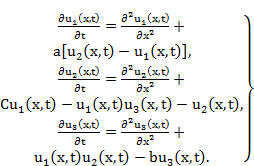(1)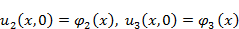,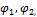andare given bounded continuous functions on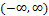,

(a, b, and c are given positive numbers).

The response system is controlled Lorenz Chaotic system as following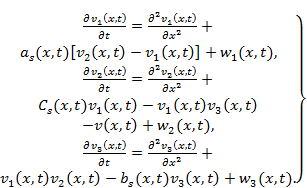(2)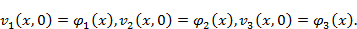Where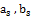and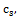of (2) are unknown functions, which need to be identified in the response system.

It is easy to see that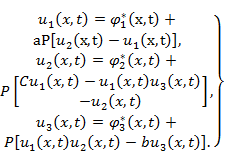(3)

Where,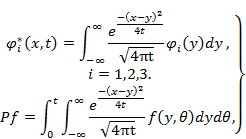Also,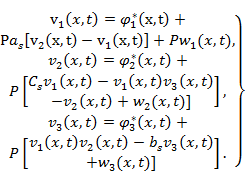(4)

Differentiating equation (3) with respect to t, one gets: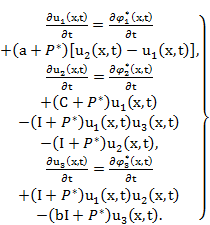(5)

Also: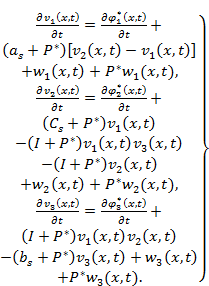(6)

Where: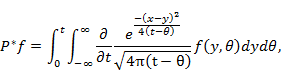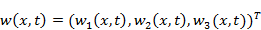is the controller which should be designed such that two systems can be Lag synchronized.

Let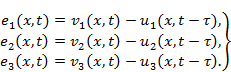(7)

Whereis the time delay for the error dynamical system. Therefor the goal of parameters identification Lag synchronization is to find an appropriate controller function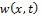and parameter adaptive lawsand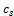, such that the synchronization errors.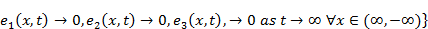(8)

and the unknown parameters.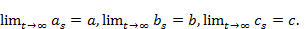(9)

3. Lag Synchronization of Lorenz Chaotic System and the Errors

In This section, we shall study the systems of errors (10) and the appearance of the lag synchronization of systems (1) and (2).

From systems (5) and (6), we get the following errors dynamical systems: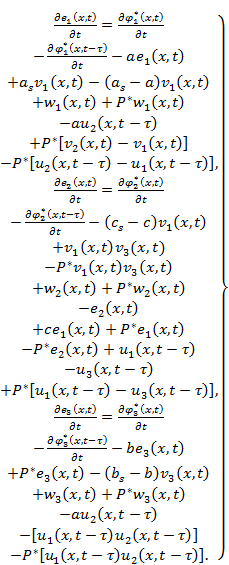(10)

Obviously, Lag synchronization of system (5) and (6) appears if the errors dynamical system (10) has an asymptotically stable equilibrium point,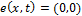, whereTheorem1. Assuming that the Lorenz chaotic system (5) derives the controlled Lorenz chaotic system (6), take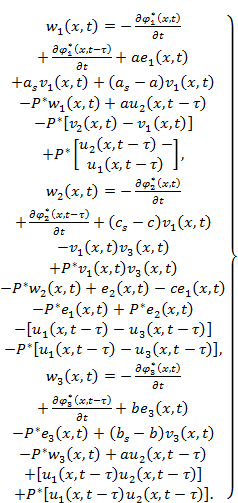(11)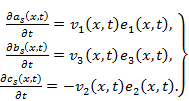(12)

Systems (5) and (6) can realize lag synchronization and the unknown confidents will be identified, i.e.; equation (8) and (9) will be achieved.

Proof Equation (10) can be converted to following form under the controller (11)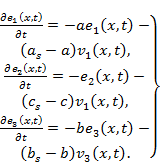(13)

Consider a Lyapunov function as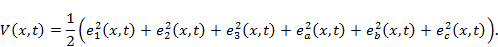Where,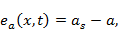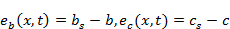.

Obviously,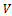is a positive definite function. Taking its time derivative along with the trajectories of equation (12) and (13) leads to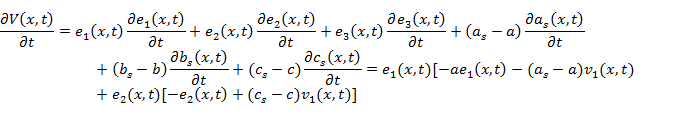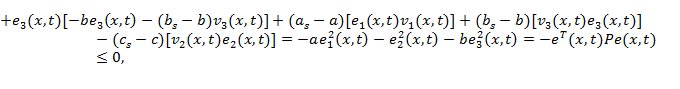Where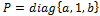. It is obvious that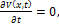if and only if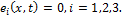Namely the set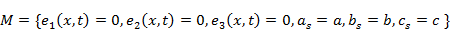is the largest invariant set contained in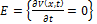for equation (13). So according to the LaSalles invariance principle , starting with arbitrary initial values of equation (13), the trajectory converges asymptotically to the set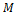,

i.e.;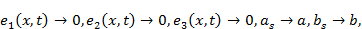and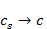as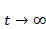.

This indicates that the lag synchronization of Lorenz chaotic system is achieved and the unknown parameters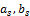and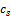can be successfully identified by using controller (11) and parameter adaptive laws (12). This completes the proof of the theorem, (Comp. [34-39].

4. Conclusion

This paper investigates the synchronization problem of coupled nonlinear diffusion systems. The lag synchronization of diffusion Lorenz chaotic system with uncertain coefficients is studied. The controller and coefficients adaptive laws are designed such that coefficient identification is realized and lag synchronization of the diffusion Lorenz system is achieved. The Lyapunov stability is also studied.

Acknowledgments

We would like to thank the referees for their careful reading of the paper and their valuable comments.

References

1. Chen, Y., Chen, X., & Chen, S. (2007). Lag Synchronization of strusturally nonequivalent chaotic systems with time delays. Nonlinear Analysis, 66, 1929_1937.
2. EL-Borai, M. M. (2004). The fundamental solutions for fractionl evolution equations of parabolic type. J. of Appl. Math. Stochastic Analysis(JAMSA), 199_211.
3. El-Borai, M. M., & F., A. (1997). On the asymptotic behavior some non-linear of parabolic systems. Memories of the faculty of science Kochi University Series A Math., 9_14.
4. EL-Borai, M. M. (1973). On stochastic differential equations in a Hilbert Space. Egyptian Statistical Journal (2).
5. EL-Borai, M. M. (1973). Partial diffferetial equations in an unbounded medium. Academia Nazionalle Dei Lincei Series VIII, 657_662.
6. El-Borai, M. M., & Assad, F. (1997). On the asymptotic behavior of some nonlinear parabolic systems. Memories of the faculty of science Kochi University Series A Math., 9_14.
7. EL-Borai, M. M., & EL-Nadi, K. (2015). Aparabolic transform and some stochastic ill-posed problems. British Journal of Mathematics and Computer Science, 9(5), 418_426.
8. EL-Borai, M. M., & EL-Nadi, K. E.-S. (2015). Fractional integrated semi groups and nonlocal fractional differential equations. Journal of Progressive Research in Mathematics(JPRM), 3(22), 553_561.
9. EL-Borai, M. M., EL-Akabawy, I. G., & EL-Nadi, K. E.-S. (2007). Approximate solutions for non-linear fractional heat equations. Int. J. Contemp. Math. Sciences, 2(27), 1307_1316.
10. EL-Borai, M. M., EL-Nadi, K. E.-S., & EL-Akabawy, I. G. (2008). On the fractional optimal control. Journal of applied Mathematics and Mechanics, 4(6), 13_18.
11. EL-Borai, M. M., EL-Nadi, K. E.-S., & Foad, H. A. (2010). On some fractional stochastic delay differential equations. Computers and Mathematics with Applications, 59, 1165_1170.
12. EL-Borai, M. M., EL-Nadi, K. E.-S., Labib, O., & M., H. (2004). Volterra equations with fractional stochastic integrals. Mathematical Problems in Engineering, 5, 453_468.
13. EL-Borai, M. M., EL-Nadi, K. E.-S., Labib, O., & M., H. (2005). Numerical method for some nonlinear stochastic differential equations. Applied Math. and Comp., 168, 65_75.
14. EL-Borai, M. M., EL-Nadin, K. E.-S., & EL-Akabawy, I. G. (2008). Fractional evolution equations with non-local conditions. Journal of Applied Mathematics and Mechanics, 4(6), 1_12.
15. EL-Boraia, M. M. (2002). Some probability densities and fundamental solution of fractional evolution equations. chaoc, Solition and Fractals(14), 433_440.
16. EL-Boraia, M. M., & Assad, F. (1997). On the cauchy problem for some partial differetial equations with time delays. Memoirs of the Faculty of Science Series A. Math.(18), 49_57.
17. EL-Boraia, M. M., & Hamza, A. (1997). An abstract dirchlet problem in the hilbert space. 4.
18. Ho, M. C., Hung, Y. C., & Chou, C. H. (2002). Phase and anti-phase synchronization of two chaotic systems by using active control. Physics Letters A, 296(1), 43_48.
19. Hu, M., & Xu, Z. (2007). Nonlinear feedback Mismatch synchronization of lorenz chaotic systems. Systems Engineering and Electronics, 29(8), 1346_1348.
20. Jia, Z. (2008). Linear generalized synchronization of chaotic systems with uncertain parameters. Journal of Systems Engineering ang Electronics, 19(4), 779_784.
21. Jia, Z., Lu, J. A., & Deng, G. M. (2007). Nonlinear state feedback and adaptive synchronization of hyperchaotic Lu systems. Systems Engineering and Electronics, 29(4), 598_600.
22. Lasall, J. P. (1960). the extent of asymptoic stability. Proceedings of the National Academy of Sciences of United States of America, 46(3), 363_365.
23. Li, C., & Liao, X. (2004). Lag synchronization of Rossler system and chua circuit via a scalar signal. Physics Letters A., 329(4-5), 301_308.
24. Li, C., Liao, X., & Wong, K. (2005). Lag synchronization of hyperchaos with Application to secure communications. Chaos, Solitons & Fractals, 23(1), 183_193.
25. Li., Z., & Xu., D. (2004). A Secure Communication Scheme using Projective Chaos Synchronization. Chaos, Solitons & Fractals, 477_481.
26. Ling, C., Wu, X., & Sun, S. (1999). A General Efficient Method for Chaotic Signal Estimation. IEEE Transactions on Signal Processing, 47, 1424_1428.
27. Lorenz, E. N. (1963). Deterministic nonperiodic Flow. Journal of the Atmospheric Sciences, 20(2), 130_141.
28. Lu, J., & Cao, J. (2005). Adaptive complete synchroization of two identical or different chaotic(hyperchaotic) systems with fully unknown parameters. Chaos, 15(4).
29. Luo, R. Z. (2008). Impulsive Control and Synchronization of a New Chaotic System. Phycics Letters A., 372, 648_653.
30. Pecora, L. M., & Carrol, T. L. (1990). Synchronization in chaotic systems. Physical Review Letters A., 64(8), 821_824.
31. Qais, H. A., & Aouda, A. A. (2011). Image Encryption Based on the General Approach for Multiple Chaotic Systems. Journal of Signal and Information Processing, 2, 238_244.
32. Wang, F., & Liu, C. (2006). A New Criterion for chaos and hyperchaoc synchronization using linear feedback control. Physics Letters A., 360(2), 274_278.
33. Yassen, M. T. (2007). Controlling, Synchronization and Tracking Chaotic Liu System using Active Backstepping Design. Physics Letters A., 372, 582_587.
34. Zhang, Q. j., Lu, j. A., & Jia, Z. (2009). Global Exponential Projective Synchronization and Lag Synchronization of Hyerchaotic Lu system. Communications in Theoretical physics, 51(4), 679_683.
35. W.G. El-Sayed, Solvability of a neutral differential equation with deviated argument, J. Math. Anal. Appl. 327 (2007), 342-350.
36. W.G. El-Sayed, On the Solvability of a Functional Integral Equation, East- West J. Math. Vol.10, No.2(2008) pp. 145-152.
37. W. G. El-Sayed, M. A. Seddeek and F. M. El-Saedy, Projection-Iteration Method for Solving Nonlinear Integral Equation of Mixed Type, Inter. J. of Research and Reviews in Applied Sciences on "Science and Mathematics with Applications" (IJRRAS), Vol. 6(3), No.19, Feb. 2011, 376-381.
38. W. G. El-Sayed, S.A El-Shehawy and E. M. El-Abd, On the Solvability of a Volterra Integral Equation of Quadratic Form in the Class of Continuous Functions, Journal of Natural Sciences and Mathematics (JNM), Qassim university, Vol.4, No. 2, Dec. 2010, pp 149-156.
39. W.G. El-Sayed and E.M.El-Abd, Monotonic solutions for a nonlinear functional integral equations of convolution type, Journal of Fixed Point Theory and Applications (JP) Vol.7, No.2, 2012, (101-111).

 Contents 1. 2. 3. 4.
Article ToolsAbstractPDF(201K)